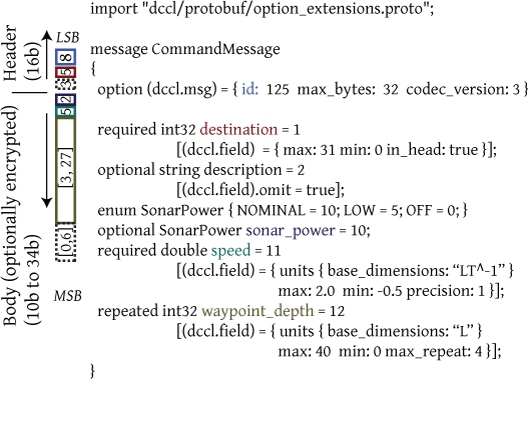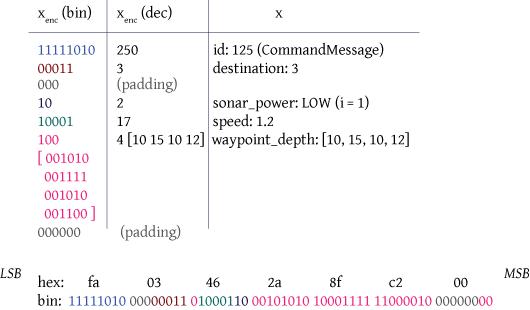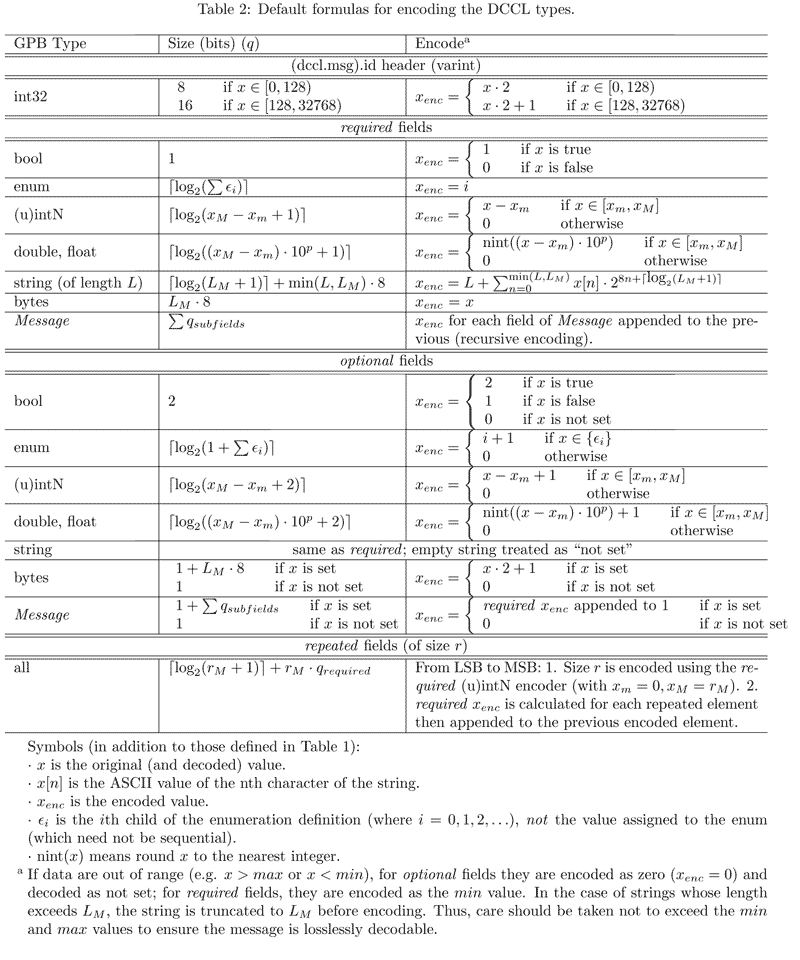DCCL v4
DCCL Encoders/Decoders (codecs)

DCCL messages are encoded and decoded using a set of "field codecs" that are responsible for encoding and decoding a given field. The library comes with a default implementation for all the Google Protocol Buffers (Protobuf) types. It is also possible to define custom field codecs to encode one or more fields of a given message (or even the entire message).

# Encoding algorithm

The following pseudo-code gives the process of encoding a DCCL message (using the dccl::v4::DefaultMessageCodec). Note this is not precisely how the actual C++ code works, but is rather given to explain the encoded message structure. Keep in mind that DCCL messages are always encoded and decoded from the least significant bit to the most significant bit.

function Encode(DCCL Message)
1: Initialize global Bits to an empty bitset
2: Encode the identifier by calling EncodeId with the value of dccl.msg.id
3: Encode the header by calling EncodeFields with all fields in the DCCL Message where in_head is true
5: Encode the body by calling in sequence
EncodeOneof with all the oneofs defined in the DCCL Message, when present
EncodeFields with all fields in the DCCL Message where in_head is false
6: Optionally encrypt the body using the header as a nonce
7: Convert Bits to a string of bytes and return this string
function EncodeId(dccl.msg.id)
1: Find the identifier codec (defaults to dccl::DefaultIdentifierCodec, otherwise specified in the dccl::Codec constructor)
2: Encode the value of dccl.msg.id using this codec and append result to Bits
function EncodeOneof(oneofs)
1: For each oneof in oneofs
2: Encode the size of oneof (equal to the number of the possible cases, including the one where no field is set) as an unsigned integer and append result to Bits.
\code
function EncodeFields(fields)
1: For each field in fields
2: Find the correct FieldCodec by the name given to dccl::FieldCodecManager::add()
If (dccl.field).codec is explicitly set use that name.
Else if this is an embedded message type and (dccl.msg).codec is explicitly set in that message definition, then use that name.
Else if (dccl.msg).codec_group is set in the root message, use that name.
Else use the name "dccl.defaultN", where (dccl.msg).codec_version = N (set in the root message or defaults to 2)
3: Encode field and append result to Bits. If field belongs to a oneof, it is encoded as a required field if and only if it is set. If field is an embedded Message, EncodeFields is called recursively with the fields of the embedded Message.
4: Append zero bits to Bits until the length of Bits is an integer number of bytes

Definitions:

1. Byte: exactly eight (8) bits
2. Field: a numbered field in the Google Protobuf Message
3. dccl::Bitset: A set of bits without byte boundaries. The front is the least significant bit, and the back is the most significant bit. Thus, appending to a Bitset means add these bits to the most significant bit.

# Example encoding

The following DCCL Message "CommandMessage" gives an example DCCL definition of a basic command, and on the left shows the parts of the encoded message. Note that LSB = least significant byte, MSB = most significant byte.Example of encoding the DCCL "CommandMessage" for a representative set of values. The table gives the unencoded $$x$$ and encoded $$x_{enc}$$ values using the formulas in Default Field Codec Reference. Below the table is the encoded message in little endian format (both in hexadecimal and binary notation).# Default Field Codec Reference

You certainly don't need to know how the fields are encoded to use DCCL, but this may be of interest to those looking to optimize their usage of DCCL or to implement custom encoders. First we will casually introduce the default encoders, then you can reference Table 2 below for precise details.

Remember that DCCL messages are always encoded and decoded from the least significant bit to the most significant bit.

## Default Identifier Codec

Each DCCL message must have a unique numeric ID (defined using (dccl.msg).id = N). To interoperate with other groups, please see http://gobysoft.org/wiki/DcclIdTable. For private work, please use IDs 124-127 (one-byte) and 128-255 (two-byte).

This ID is used in lieu of the DCCL message name on the wire. It is encoded using a one- or two-byte value, allowing for a larger set of values to be used than a single byte would allow, but still preserving a set of one-byte identifiers for frequently sent messages. This is always the first 8 or 16 bits of the message so that the dccl::Codec knows which message to decode.

The first (least significant) bit of the ID determines if it is a one- or two-byte identifier. If the first bit is true, it's a two-byte identifier and the next 15 bits give the actual ID. If the first bit is false, the next 7 bits give the ID.

Two examples:

1. One byte ID [0-128): (dccl.msg).id = 124. Decimal 124 is binary 1111100, which is appended to a single 0 bit to indicate a one-byte ID. Thus, the ID on the wire is 11111000 (or 124*2 = 248)
2. Two byte ID [128-32768): (dccl.msg).id = 240. Decimal 240 is binary 0000000 11110000. This is appended to a single 1 bit to indicate a two-byte ID, giving an encoded ID of 00000001 11100001 (or 240*2+1 = 481).

## Default Numeric Codec

Numeric values are all encoded essentially the same way. Integers are treated as floating point values with zero precision. Precision is defined as the number of decimal places to preserve (e.g. precision = 3 means round to the closest thousandth, precision = -1 means round to the closest tens). Thus, integer fields can also have negative precision, if desired. Fields are bounded by a minimum and maximum allowable value, based on the underlying source of the data.

To encode, the numeric value is rounded to the desired precision, and then multiplied by the appropriate power of ten to make it an integer. Then it is increased or decreased so that zero (0) represents the minimum encodable value. At this point, it is simply an unsigned integer. To encode the optional field's "not set", an additional value (not an additional bit) is reserved. To allow "not set" to be the zero (0) encoded value, all other values are incremented by one.

This default encoder assumes unset fields are rare. If you commonly have unset optional fields, you may want to implement a "presence bit" encoder that uses a separate bit to indicate if a field is set or not. These are two extremes of the more general purpose idea of an entropy encoder, such as the arithmetic encoder. In that case, "not set" is simply another symbol that has a probability mass relative to the actual values to capture the frequency with which fields are set or not set.

For example:

required double x = 1 [(dccl.field) = { min: -10000 max: 10000 precision: 1 }];

The field takes 18 bits: $$\lceil \hbox{log}_2(10000-(-10000)\cdot 10^1 + 1) \rceil =\lceil 17.61 \rceil = 18$$.

Say we wanted to encode the value 10.56:

• Round to tenths: 10.6
• Subtract the minimum value: 10.6 - (-10000) = 10010.6
• Multiply by precision orders of magnitude: 10010.6*10 = 100106 = binary 01 10000111 00001010

## Default Enumeration Codec

Enumerations are treated like unsigned integers, where the enumeration keys are given values based on the order they are declared (not the value given in the .proto file).

For example:

enum VehicleClass { AUV = 10; USV = 5; SHIP = 3; }
optional VehicleClass veh_class = 4;

In this case (for encoding): AUV is 0, USV is 1, SHIP is 2. After this mapping, the field is encoded exactly like an equivalent integer field (with max = 2, min = 0 in this case).

## Default Boolean Codec

Booleans are simple. If they are required, they are encoded with false = 0, true = 1. If they are optional, they are tribools with "not set" = 0, false = 1, true = 2

## Default String Codec

Strings are given a maximum size in the proto file (max_length). A small integer (minimally sized like a required unsigned int field to encode 0 to max_length) is included first to specify the length of the following string. Then the string is encoded using the basic one-byte character values (ASCII).

For example:

optional string message = 4 [(dccl.field).max_length = 10];

Say we want to encode "HELLO":

• The string is length 5, so we insert 4 bits ( $$\lceil \hbox{log}_2(10 + 1) \rceil = 4$$) with value 5: 0101
• Following, we add the ASCII values: 01001111 01001100 01001100 01000101 01001000
• The full encoded value is thus 0100 11110100 11000100 11000100 01010100 10000101

## Default Bytes Codec

Like the string codec, but not variable length. It always takes max_length bytes in the message, and if it is optional, a presence bit is added at the front. If the presence bit is false, the bytes are omitted.

## Default Message Codec

Sub-messages are encoded recursively. In the case of an optionanl message, a presence bit is added before the message fields. If the presence bit is false (indicating the message is not set), no further bits are used.

## Mathematical formulas for Default Field Codecs

See Table 1 in the DCCL Interface Descriptor Language (IDL) for symbol definitions. The formulas below in Table 2 refer to DCCLv3 defaults (i.e. codec_version = 3 which is equivalent to codec = "dccl.default3"). A few things that may make it easier to read this table:

• Left bit-shifting by N bits ( $$x << N$$) is equivalent to multiplying by powers of two to the N ( $$x \cdot 2^N$$).
• $$\lceil \hbox{log}_2(b-a+1) \rceil$$ is the fewest number of bits that can hold all the values between $$a$$ and $$b$$. For example, $$a=0$$ through $$b=7$$ can be stored in 3 ( $$\lceil \hbox{log}_2(7-0+1) \rceil$$) bits. Even if $$b$$ was 4, 5, or 6, it would still take 3 bits since you cannot have partial bits, hence the ceiling function ( $$\lceil \ldots \rceil$$).
• Appending bits in this context means adding these bits to the most signficant end (the left side if the bits are written MSB to LSB from left to right). For example, appending a Bitset of size 2 with decimal value 3 (b11) to a Bitset of size 3 with decimal value 1 (b001) would yield a Bitset of size 5 with value 25 (b11001). This is equivalent to the mathematical expression $$1+3\cdot 2^3 = 25$$# Custom Field Codecs

1. subclass one of the base classes in the API classes for defining new custom field encoders/decoders
2. add the class into the dccl::FieldCodecManager with a given string name
3. set a given field's (dccl.field).codec to the name given to dccl::FieldCodecManager, or the (dccl.msg).codec to change the entire message's field codec.